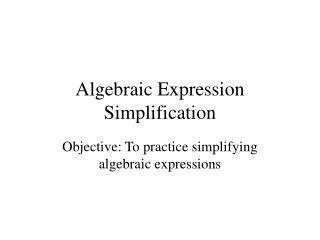DownloadDownload PresentationAlgebraic Expression Simplification

# Algebraic Expression Simplification

Télécharger la présentation## Algebraic Expression Simplification

- - - - - - - - - - - - - - - - - - - - - - - - - - - E N D - - - - - - - - - - - - - - - - - - - - - - - - - - -
##### Presentation Transcript

1. Algebraic Expression Simplification Objective: To practice simplifying algebraic expressions

2. Directions: Restate each expression using the distributive property.

3. 3(11 + 12)

4. 6t + 11t

5. 2r + 12s

6. (9 + 8)v

7. (4x + 9y)2

8. 3ab + 6a

9. 4(x + 3)

10. 2(6 + 9)

11. Directions: Rewrite each expression using a commutative property. Do not simplify.

12. 5 + 9

13. 9 + 18y

14. A + B

15. 8 + 4h

16. 0 + 22

17. 5c + 9d

18. 15 + 19

19. 5m + 9n

20. 25 + 19

21. Directions: Rewrite each expression using a associative property. Do not simplify.

22. (b . 6) . 5

23. 25 + (19 + m)

24. (P . 7) . 4

25. n . (6 . 5)

26. (15 + 4w) + w

27. a(b0)

28. 7(6z)

29. 2 + (5 + 1)

30. (2 + 3) + 19

31. Directions: Rewrite each expression using the identity property of addition, identity property of multiplication, or the multiplicative property of zero.

32. b . 0

33. b + 0

34. 0 + 1

35. 1 . 0

36. Q . 1

37. 1 . azb

38. azb . 0

39. (6 . 5) + 0

40. (b . 6) . 1